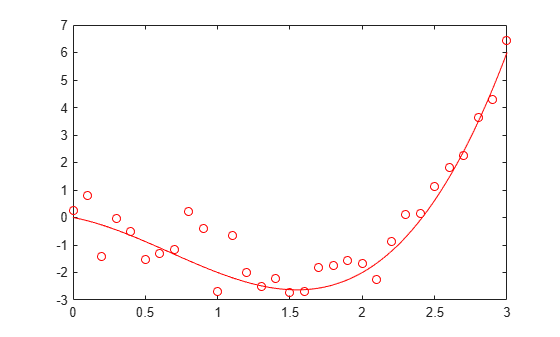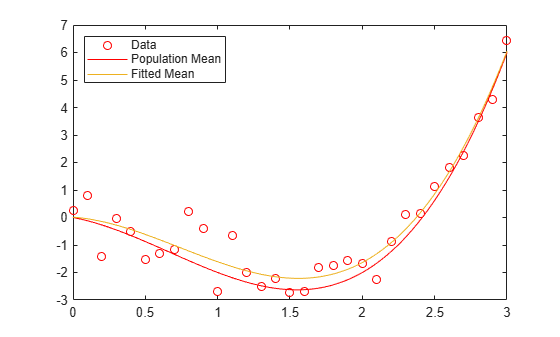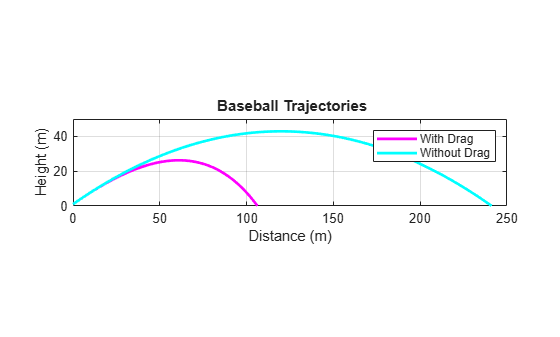# refcurve

## Syntax

refcurve(p) refcurve refcurve(ax,p) hcurve = refcurve(...) 

## Description

refcurve(p) adds a polynomial reference curve with coefficients p to the current axes. If p is a vector with n+1 elements, the curve is:

y = p(1)*x^n + p(2)*x^(n-1) + ... + p(n)*x + p(n+1)

refcurve with no input arguments adds a line along the x axis.

refcurve(ax,p) uses the plot axes specified in ax, an Axes object. For more information, see axes.

hcurve = refcurve(...) returns the handle hcurve to the curve using any of the input argument combinations in the previous syntaxes.

## Examples

collapse all

Generate data with a polynomial trend.

p = [1 -2 -1 0]; t = 0:0.1:3; rng default % For reproducibility y = polyval(p,t) + 0.5*randn(size(t));

Plot data and add the population mean function using refcurve .

plot(t,y,'ro') h = refcurve(p); h.Color = 'r';Also add the fitted mean function.

q = polyfit(t,y,3); refcurve(q) legend('Data','Population Mean','Fitted Mean',... 'Location','NW')Introduce the relevant physical constants.

M = 0.145; % Mass (kg) R = 0.0366; % Radius (m) A = pi*R^2; % Area (m^2) rho = 1.2; % Density of air (kg/m^3) C = 0.5; % Drag coefficient D = rho*C*A/2; % Drag proportional to the square of the speed g = 9.8; % Acceleration due to gravity (m/s^2)

Simulate the trajectory with drag proportional to the square of the speed, assuming constant acceleration in each time interval.

dt = 1e-2; % Simulation time interval (s) r0 = [0 1]; % Initial position (m) s0 = 50; % Initial speed (m/s) alpha0 = 35; % Initial angle (deg) v0 = s0*[cosd(alpha0) sind(alpha0)]; % Initial velocity (m/s) r = r0; v = v0; trajectory = r0; while r(2) > 0 a = [0 -g] - (D/M)*norm(v)*v; v = v + a*dt; r = r + v*dt + (1/2)*a*(dt^2); trajectory = [trajectory;r]; end

Plot trajectory and use refcurve to add the drag-free parabolic trajectory (found analytically) to the plot of trajectory.

figure plot(trajectory(:,1),trajectory(:,2),'m','LineWidth',2) xlim([0,250]) h = refcurve([-g/(2*v0(1)^2),... (g*r0(1)/v0(1)^2) + (v0(2)/v0(1)),... (-g*r0(1)^2/(2*v0(1)^2)) - (v0(2)*r0(1)/v0(1)) + r0(2)]); h.Color = 'c'; h.LineWidth = 2; axis equal ylim([0,50]) grid on xlabel('Distance (m)') ylabel('Height (m)') title('{\bf Baseball Trajectories}') legend('With Drag','Without Drag')## Version History

Introduced before R2006a